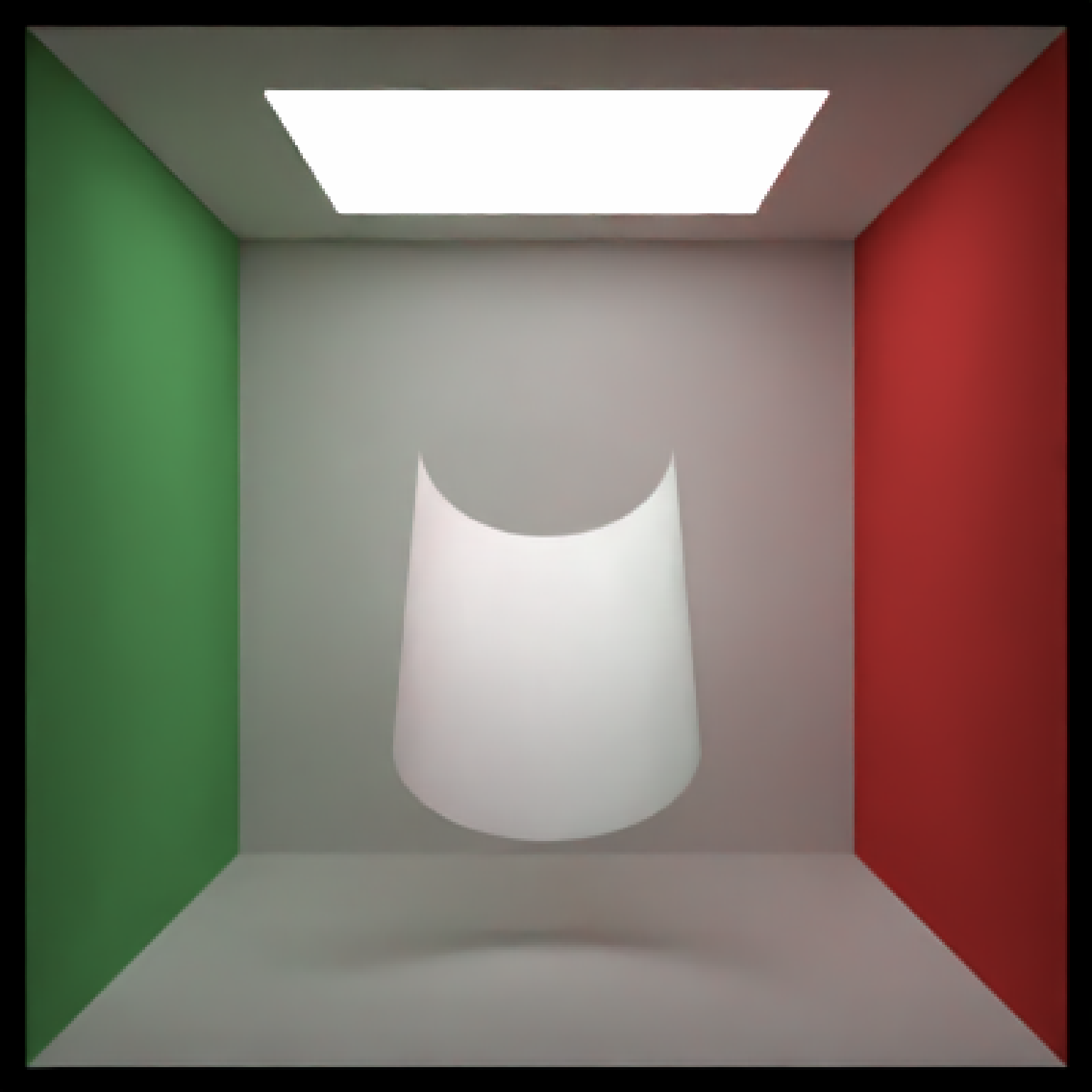Cylinder Object

cylinder(
x = 0,
y = 0,
z = 0,
length = 1,
phi_min = 0,
phi_max = 360,
material = diffuse(),
angle = c(0, 0, 0),
order_rotation = c(1, 2, 3),
flipped = FALSE,
scale = c(1, 1, 1),
capped = TRUE
)

## Arguments

x

Default 0. x-coordinate of the center of the cylinder

y

Default 0. y-coordinate of the center of the cylinder

z

Default 0. z-coordinate of the center of the cylinder

Default 1. Radius of the cylinder.

length

Default 1. Length of the cylinder.

phi_min

Default 0. Minimum angle around the segment.

phi_max

Default 360. Maximum angle around the segment.

material

Default diffuse.The material, called from one of the material functions diffuse, metal, or dielectric.

angle

Default c(0, 0, 0). Angle of rotation around the x, y, and z axes, applied in the order specified in order_rotation.

order_rotation

Default c(1, 2, 3). The order to apply the rotations, referring to "x", "y", and "z".

flipped

Default FALSE. Whether to flip the normals.

scale

Default c(1, 1, 1). Scale transformation in the x, y, and z directions. If this is a single value, number, the object will be scaled uniformly.

capped

Default TRUE. Whether to add caps to the segment. Turned off when using the light() material. Note: emissive objects may not currently function correctly when scaled.

## Value

Single row of a tibble describing the cylinder in the scene.

## Examples

#Generate a cylinder in the cornell box. Add a cap to both ends.

if(run_documentation()) {
generate_cornell() %>%
add_object(cylinder(x = 555/2, y = 250, z = 555/2,
length = 300, radius = 100, material = metal())) %>%
render_scene(lookfrom = c(278, 278, -800) ,lookat = c(278, 278, 0), fov = 40,
ambient_light = FALSE, samples = 128, parallel = TRUE, clamp_value = 5)
}#Rotate the cylinder
if(run_documentation()) {
generate_cornell() %>%
add_object(cylinder(x = 555/2, y = 250, z = 555/2,
length = 300, radius = 100, angle = c(0, 0, 45),
material = diffuse())) %>%
render_scene(lookfrom = c(278, 278, -800) ,lookat = c(278, 278, 0), fov = 40,
ambient_light = FALSE, samples = 128, parallel = TRUE, clamp_value = 5)
}# Only render a subtended arc of the cylinder,
if(run_documentation()) {
generate_cornell(lightintensity=3) %>%
add_object(cylinder(x = 555/2, y = 250, z = 555/2, capped = FALSE,
length = 300, radius = 100, angle = c(45, 0, 0), phi_min = 0, phi_max = 180,
material = diffuse())) %>%
render_scene(lookfrom = c(278, 278, -800) ,lookat = c(278, 278, 0), fov = 40,
ambient_light = FALSE, samples = 128, parallel = TRUE, clamp_value = 5)
}